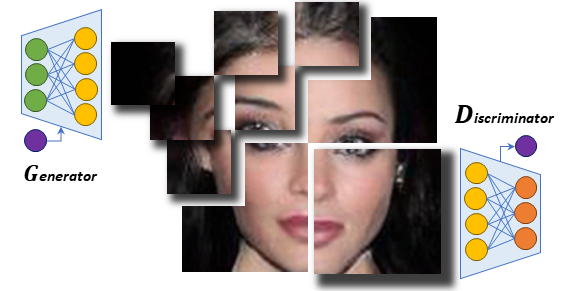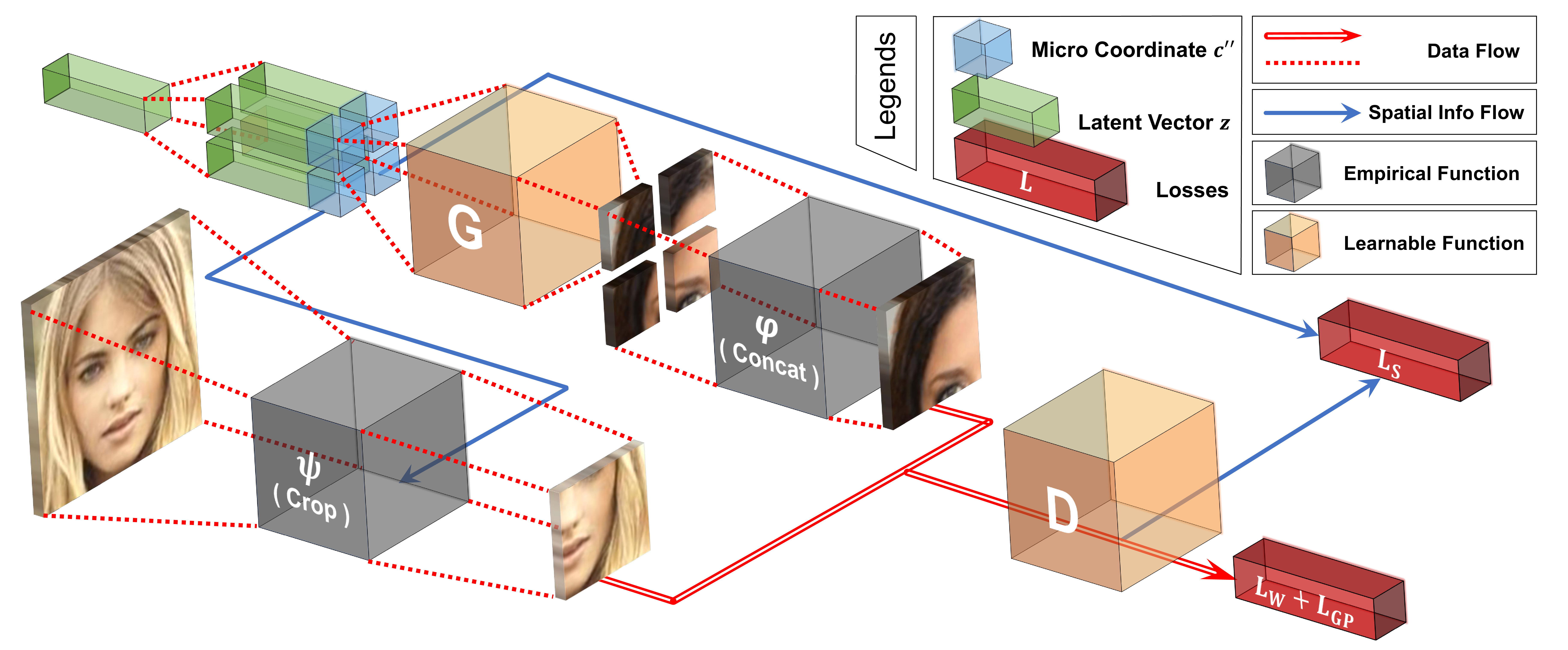# ICCV 2019: Image Synthesis(Part 1)

## Identity From Here, Pose From There: Self-Supervised Disentanglement and Generation of Objects Using Unlabeled VideosThe proposed model takes as input an ID image and a pose image, and generates an output image with the identity of the ID image and the pose of the pose image.

### MethodsThe generator takes as input both the identity reference image $I_{id}$ and the pose reference image $I_{pose}$, and tries to generate an output image that matches $I_{target}$ , which has the same identity as $I_{id}$ but with the pose of $I_{pose}$ . Notice how the pose encoded feature (yellow block) is used to generate both $I_{target}$ and $I_{pose}$ , so it cannot contain any identity information. Likewise, the identity encoded feature (green block) is used to generate both $I_{target}$ and $I_{id}$ , so it cannot contain any pose information. Furthermore, we propose a novel pixel verification module (PVM, details shown on the right) which computes a verifiability score between Ig and $I_{id}$ , indicating the extent to which pixels in $I_g$ can be traced back to $I_{id}$.

### Constructing ID-pose-target training tripletsThe authors first sample two images from the same video clip as $I_{id}$ and $I_{target}$. The assumption is that these images will contain the same object instance, which is generally true for short clips (for long videos, unsupervised tracking could also be applied). They then retrieve a nearest neighbor of $I_{target}$ from other videos (so that it’s unlikely to have the same identity) using a pre-trained convnet, to serve as the pose reference image $I_{pose}$.

## Unsupervised Robust Disentangling of Latent Characteristics for Image SynthesisLeft: synthesizing a new image x2 that exhibits the apperance of x1 and pose of x3. Right: training our generative model using pairs of images with same/different appearance. T and T ′ estimate the mutual information between π and α. The gradients of T are used to guide disentanglement, while T ′ detects overpowering of T and estimates γ to counteract it.

## COCO-GAN: Generation by Parts via Conditional Coordinating(Oral)

 Project Page Code(TensorFlow)The authors propose COnditional COordinate GAN (COCO-GAN) of which the generator generates images by parts based on their spatial coordinates as the condition. On the other hand, the discriminator learns to justify realism across multiple assembled patches by global coherence, local appearance, and edge-crossing continuity.For the COCO-GAN training, the latent vectors are duplicated multiple times, concatenated with micro coordinates, and feed to the generator to generate micro patches. Then we concatenate multiple micro patches to form a larger macro patch. The discriminator learns to discriminate between real and fake macro patches and an auxiliary task predicting the coordinate of the macro patch. Notice that none of the models requires full images during training.During the testing phase, the micro patches generated by the generator are directly combined into a full image as the final output. Still, none of the models requires full images. Furthermore, the generated images are high-quality without any post-processing in addition to a simple concatenation.

Full notes with code: COCO-GAN: Generation by Parts via Conditional Coordinating - ICCV 2019

## Seeing What a GAN Cannot Generate(Oral)(a) We compare the distribution of object segmentations in the training set of LSUN churches to the distribution in the generated results: objects such as people, cars, and fences are dropped by the generator.

(b) We compare pairs of a real image and its reconstruction in which individual instances of a person and a fence cannot be generated. In each block, we show a real photograph (top-left), a generated re- construction (top-right), and segmentation maps for both (bottom).

### Generated Image Segmentation Statistics

The authors characterize omissions in the distribution as a whole, using Generated Image Segmentation Statistics: segment both generated and ground truth images andcompare the distributions of segmented object classes. For example, the above figure shows that in a church GAN model,object classes such as people, cars, and fences appear on fewer pixels of the generated distribution as compared to the training distribution.

#### Defining Fréchet Segmentation Distance (FSD)

It is an interpretable analog to the popular Fréchet Inception Distance (FID) metric:

In FSD formula, $\mu_{t}$ is the mean pixel count for each object class over a sample of training images, and $\Sigma_{t}$ is the covariance of these pixel counts. Similarly, $\mu_{g}$ and $\Sigma_{g}$ reflect segmentation statistics for the generative model.

### Layer Inversion

Once omitted object classes are identified, the author want to visualize specific examples of failure cases. To do so, they must find image instances where the GAN should generate an object class but does not. We find such cases using a new reconstruction method called Layer Inversion which relaxes reconstruction to a tractable problem. Instead of inverting the entire GAN, their method inverts a layer of the generator.First, train a network E to invert G; this is used to obtain an initial guess of the latent $z_0 = E(x)$ and its intermediate representation $r_0 = g_n (· · · (g_1 (z_0)))$. Then $r_0$ is used to initialize a search for $r^∗$ to obtain a reconstruction $x′ = G_f (r^∗)$ close to the target x.

Full notes with code: Seeing What a GAN Cannot Generate - Bau - ICCV 2019

## Image2StyleGAN: How to Embed Images Into the StyleGAN Latent Space?(Oral)

### Embedding Algorithm to StyleGAN Latent Space

Starting from a suitable initialization w, we search for an optimized vector $w^∗$ that minimizes the loss function that measures the similarity between the given image and the image generated from $w^∗$.Loss function use VGG-16 perceptual loss and pixel-wise MSE loss:

### Latent Space Operations and Applications

The authors propose to use three basic operations on vectors in the latent space: linear interpolation, crossover, and adding a vector and a scaled difference vector. These operations correspond to three semantic image processing applications: morphing, style transfer, and expression transfer.

Expression transfer results: